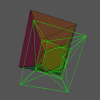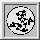To: ProgramsOptionsOutputFormatsGeomviewPrintQhullPrecisionTraceFunctions (local)

#Qhull output options

This section lists the output options for Qhull. These options are indicated by lower case characters. See Formats, Print, and Geomview for other output options.

## Output options

Qhull prints its output to standard out. All output is printed text. The default output is a summary (option 's'). Other outputs may be specified as follows.

f
print all fields of all facets
i
print vertices incident to each facet
m
print Mathematica output (2-d and 3-d)
n
print hyperplane normals with offsets
o
print OFF file format (dim, points and facets)
p
print vertex and point coordinates
s
print summary to stderr

Related options
F
Ft
G
Geomview output
P
Print options

### »f - print all fields of all facets

Print all fields of facetT for all facets. The facet is the primary data structure for Qhull.

Option 'f' is for debugging. Most of the fields are available via the 'F' options. If you need specialized information from Qhull, you can use the Qhull library or C++ interface.

Use the 'FF' option to print the facets but not the ridges.

### »i - print vertices incident to each facet

The first line is the number of facets. The remaining lines list the vertices for each facet, one facet per line. The indices are 0-relative indices of the corresponding input points. The facets are oriented. Option 'Fv' displays an unoriented list of vertices with a vertex count per line. Options 'o' and 'Ft' displays coordinates for each vertex prior to the vertices for each facet.

Simplicial facets (e.g., triangles in 3-d) consist of d vertices. Non-simplicial facets in 3-d consist of 4 or more vertices. For example, a facet of a cube consists of 4 vertices. Use option 'Qt' to triangulate non-simplicial facets.

For 4-d and higher convex hulls and 3-d and higher Delaunay triangulations, d vertices are listed for all facets. A non-simplicial facet is triangulated with its centrum and each ridge. The index of the centrum is higher than any input point. Use option 'Fv' to list the vertices of non-simplicial facets as is. Use option 'Ft' to print the coordinates of the centrums as well as those of the input points. The centrum indices for option 'i' are one more than the centrum indices for option 'Ft'.

### »m - print Mathematica output

Qhull writes a Mathematica file for 2-d and 3-d convex hulls, 2-d and 3-d halfspace intersections, and 2-d Delaunay triangulations. Qhull produces a list of objects that you can assign to a variable in Mathematica, for example: "list= << <outputfilename> ". If the object is 2-d, it can be visualized by "Show[Graphics[list]] ". For 3-d objects the command is "Show[Graphics3D[list]] ". Now the object can be manipulated by commands of the form "Show[%, <parametername> -> <newvalue>]".

For Delaunay triangulation orthogonal projection is better. This can be specified, for example, by "BoxRatios: Show[%, BoxRatios -> {1, 1, 1e-8}]". To see the meaningful side of the 3-d object used to visualize 2-d Delaunay, you need to change the viewpoint: "Show[%, ViewPoint -> {0, 0, -1}]". By specifying different viewpoints you can slowly rotate objects.

For halfspace intersections, Qhull produces the dual convex hull.

See Is Qhull available for Mathematica? for URLs.

### »n - print hyperplane normals with offsets

The first line is the dimension plus one. The second line is the number of facets. The remaining lines are the normals for each facet, one normal per line. The facet's offset follows its normal coefficients.

The normals point outward, i.e., the convex hull satisfies Ax <= -b where A is the matrix of coefficients and b is the vector of offsets.

A point is inside or below a hyperplane if its distance to the hyperplane is negative. A point is outside or above a hyperplane if its distance to the hyperplane is positive. Otherwise a point is on or coplanar to the hyperplane.

If cdd output is specified ('FD'), Qhull prints the command line, the keyword "begin", the number of facets, the dimension (plus one), the keyword "real", and the normals for each facet. The facet's negative offset precedes its normal coefficients (i.e., if the origin is an interior point, the offset is positive). Qhull ends the output with the keyword "end".

### »o - print OFF file format

The output is:

• The first line is the dimension
• The second line is the number of points, the number of facets, and the number of ridges.
• All of the input points follow, one per line.
• Then Qhull prints the vertices for each facet. Each facet is on a separate line. The first number is the number of vertices. The remainder is the indices of the corresponding points. The vertices are oriented in 2-d, 3-d, and in simplicial facets.

Option 'Ft' prints the same information with added points for non-simplicial facets.

Option 'i' displays vertices without the point coordinates. Option 'p' displays the point coordinates without vertex and facet information.

In 3-d, Geomview can load the file directly if you delete the first line (e.g., by piping through 'tail +2').

For Voronoi diagrams (qvoronoi), option 'o' prints Voronoi vertices and Voronoi regions instead of input points and facets. The first vertex is the infinity vertex [-10.101, -10.101, ...]. Then, option 'o' lists the vertices in the Voronoi region for each input site. The regions appear in site ID order. In 2-d, the vertices of a Voronoi region are sorted by adjacency (non-oriented). In 3-d and higher, the Voronoi vertices are sorted by index. See the 'FN' option for listing Voronoi regions without listing Voronoi vertices.

If you are using the Qhull library, options 'v o' have the side effect of reordering the neighbors for a vertex.

### »p - print vertex and point coordinates

The first line is the dimension. The second line is the number of vertices. The remaining lines are the vertices, one vertex per line. A vertex consists of its point coordinates

With the 'Gc' and 'Gi' options, option 'p' also prints coplanar and interior points respectively.

For qvoronoi, it prints the coordinates of each Voronoi vertex.

For qdelaunay, it prints the input sites as lifted to a paraboloid. For qhalf it prints the dual points. For both, option 'p' is the same as the first section of option 'o'.

Use 'Fx' to list the point ids of the extreme points (i.e., vertices).

If a subset of the facets is selected ('Pdk', 'PDk', 'Pg' options), option 'p' only prints vertices and points associated with those facets.

If cdd-output format is selected ('FD'), the first line is "begin". The second line is the number of vertices, the dimension plus one, and "real". The vertices follow with a leading "1". Output ends with "end".

### »s - print summary to stderr

The default output of Qhull is a summary to stderr. Options 'FS' and 'Fs' produce the same information for programs. Note: Windows 95 and 98 treats stderr the same as stdout. Use option 'TO file' to separate stderr and stdout.

The summary lists the number of input points, the dimension, the number of vertices in the convex hull, and the number of facets in the convex hull. It lists the number of selected ("good") facets for options 'Pg', 'Pdk', qdelaunay, or qvoronoi (Delaunay triangulations only use the lower half of a convex hull). It lists the number of coplanar points. For Delaunay triangulations without 'Qc', it lists the total number of coplanar points. It lists the number of simplicial facets in the output.

The terminology depends on the output structure.

The summary lists these statistics:

• number of points processed by Qhull
• number of hyperplanes created
• number of distance tests (not counting statistics, summary, and checking)
• number of merged facets (if any)
• number of distance tests for merging (if any)
• CPU seconds to compute the hull
• the maximum joggle for 'QJ'
or, the probability of precision errors for 'QJ TRn'
• total area and volume (if computed, see 'FS' 'FA' 'Fa' 'PAn')
• max. distance of a point above a facet (if non-zero)
• max. distance of a vertex below a facet (if non-zero)

The statistics include intermediate hulls. For example 'rbox d D4 | qhull' reports merged facets even though the final hull is simplicial.

Qhull starts counting CPU seconds after it has read and projected the input points. It stops counting before producing output. In the code, CPU seconds measures the execution time of function qhull() in libqhull.c. If the number of CPU seconds is clearly wrong, check qh_SECticks in user.h.

The last two figures measure the maximum distance from a point or vertex to a facet. They are not printed if less than roundoff or if not merging. They account for roundoff error in computing the distance (c.f., option 'Rn'). Use 'Fs' to report the maximum outer and inner plane.

A number may appear in parentheses after the maximum distance (e.g., 2.1x). It is the ratio between the maximum distance and the worst-case distance due to merging two simplicial facets. It should be small for 2-d, 3-d, and 4-d, and for higher dimensions with 'Qx'. It is not printed if less than 0.05.The Geometry Center Home Page